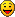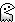Uniformly Distributed Random Numbers

Post

Uniformly Distributed Random Numbers

#1
I just found this good article, with diagrams and code, on using rejection sampling to obtain uniformly distributed integers in a given range from a random number generator.
Post

Re: Uniformly Distributed Random Numbers

#2
Interesting read, thanks for linking. I'd be interested to see use cases where this actually provides a noticeably fairer outcome than the standard double-precision algorithm (which, as he mentions, has a relative error of 2^-53).

In LT the 2^-53 error has turned out to be good enoughIn fact, with such an incredibly tiny error, I would be more concerned about the random source being well-behaved. My intuition says that, in order for that error to show up as a bias, the random generator would have to be really good (I'm sure you could quantify exactly what that means using the error bound -- e.g., how much could a uniform-bit-generator diverge from perfect uniformity before the quantization error would be secondary to the generator's uniformity error?)

Rejection sampling is the bomb. Sometimes a really expensive bomb, but the bomb nonetheless